#DAY 5
0
Modern Web

## Day 5— 自動化回信機(2) 讀取試算表內容

``````function onEdit() {
console.log('我被改變了喔! ')
}
``````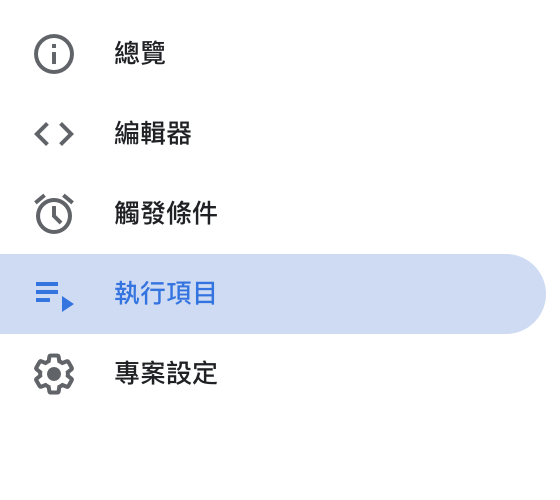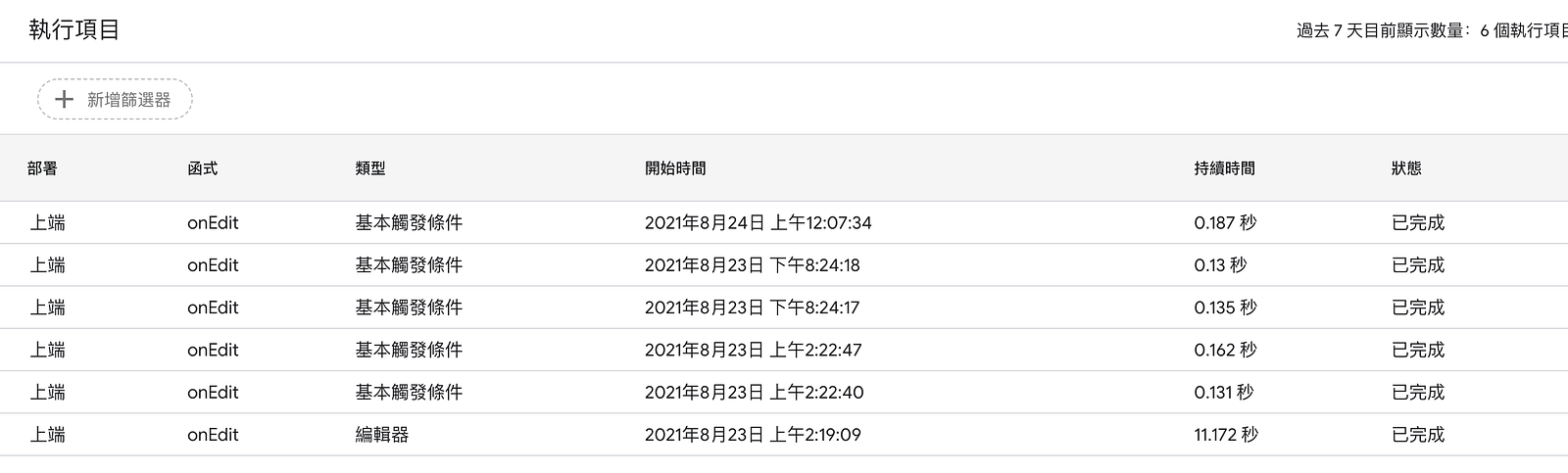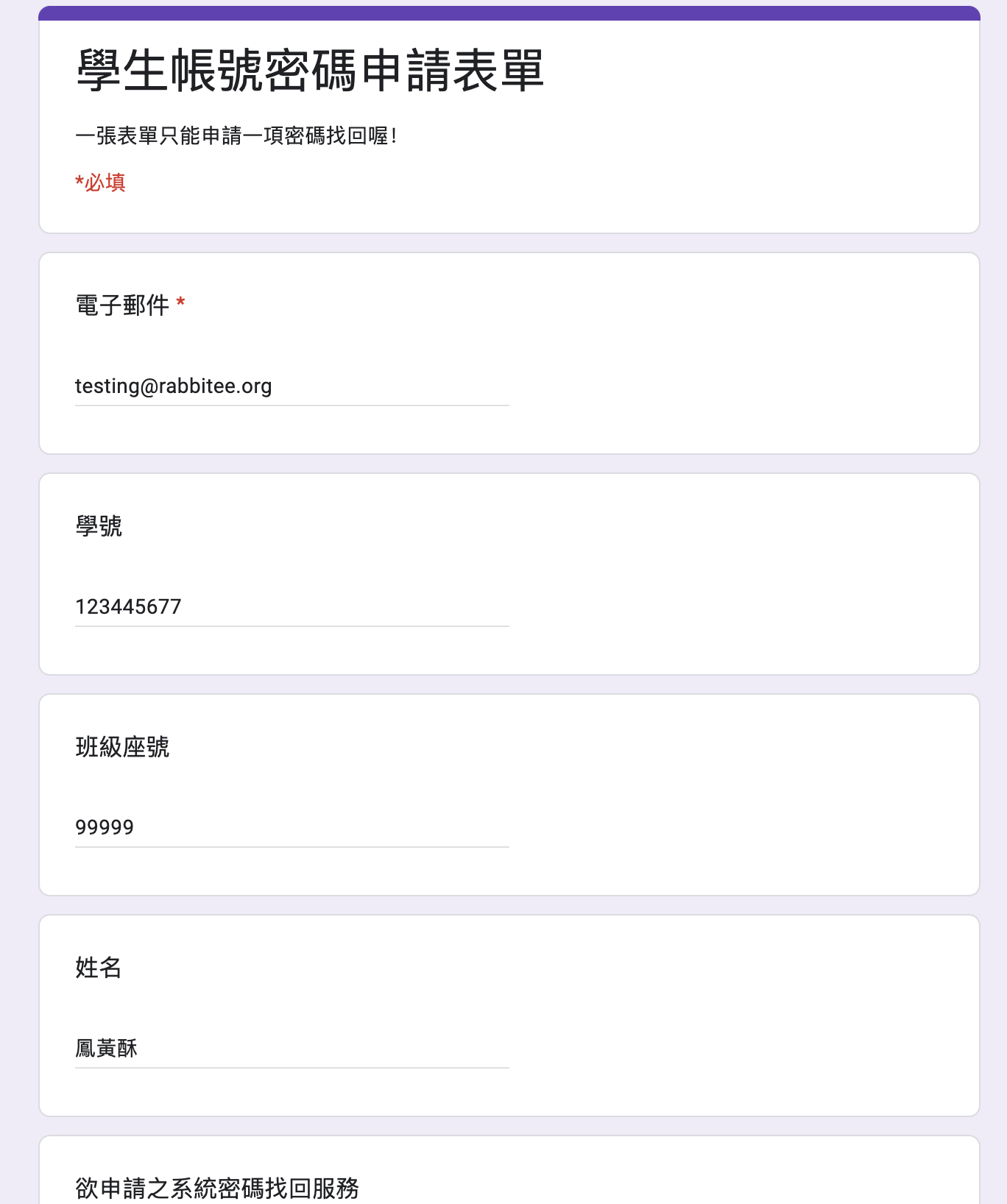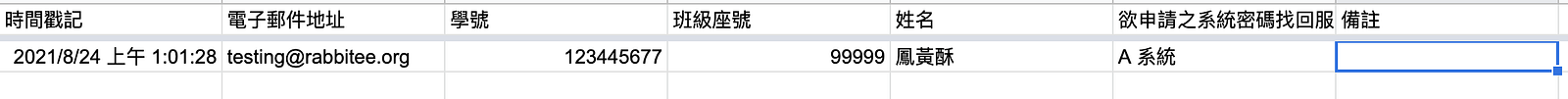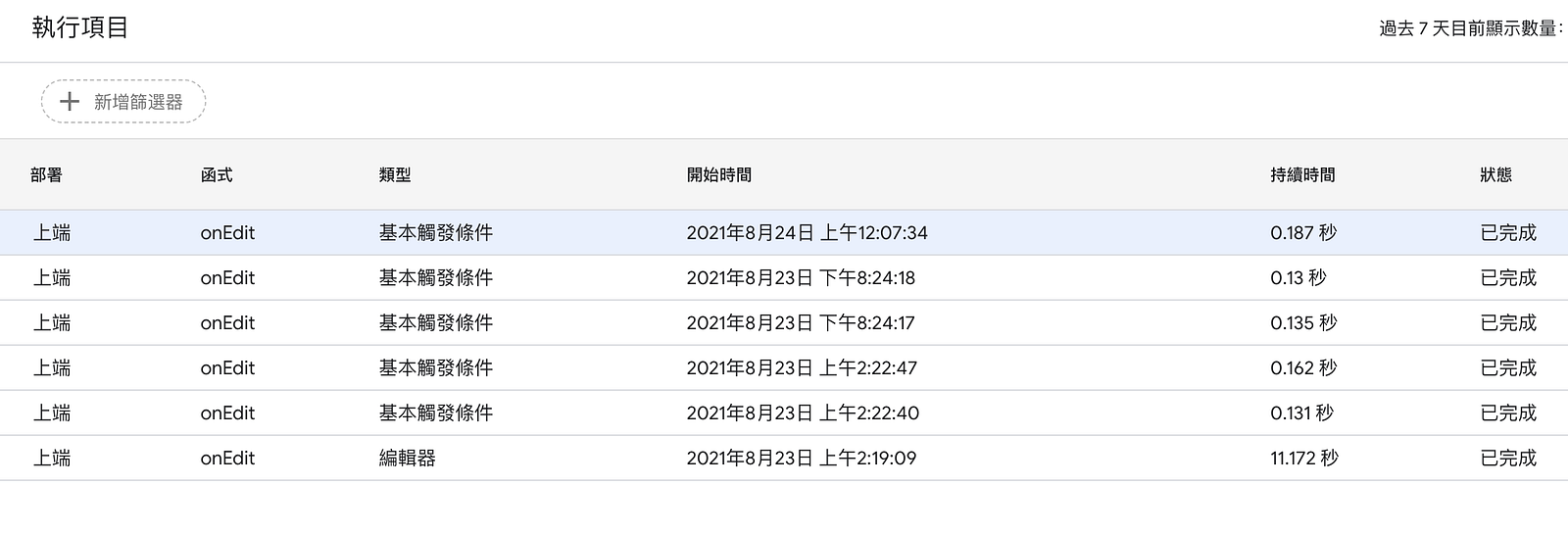#### 所以問題的答案出來囉～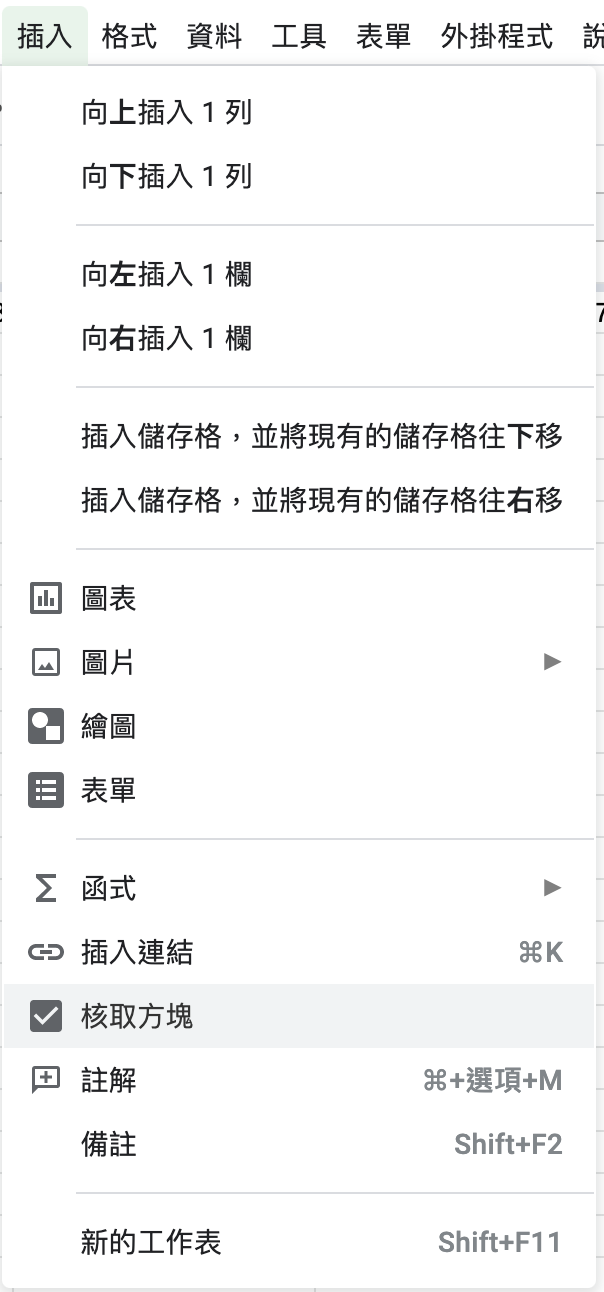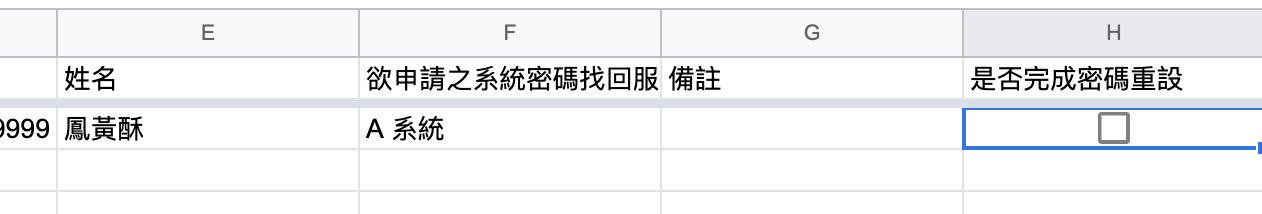#### 應該也人會問，那麼後面我每一格都要自己慢慢加嗎？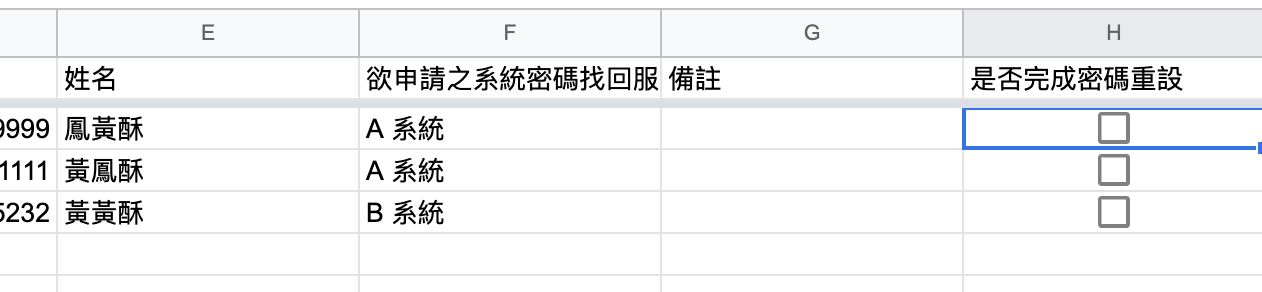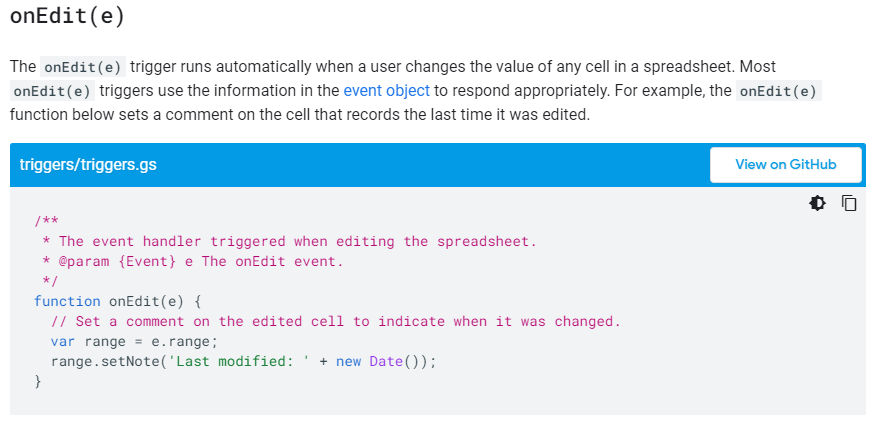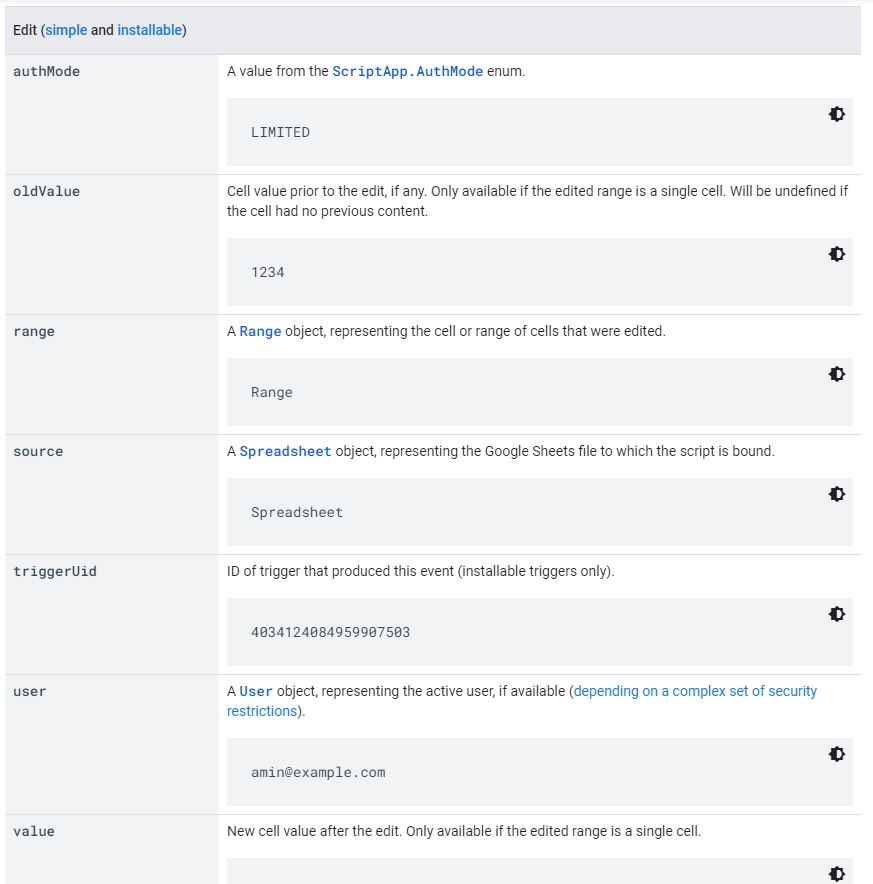range 回傳的是一個 `Range` 物件 `(註一)`，代表的是該 event 在表單中位置。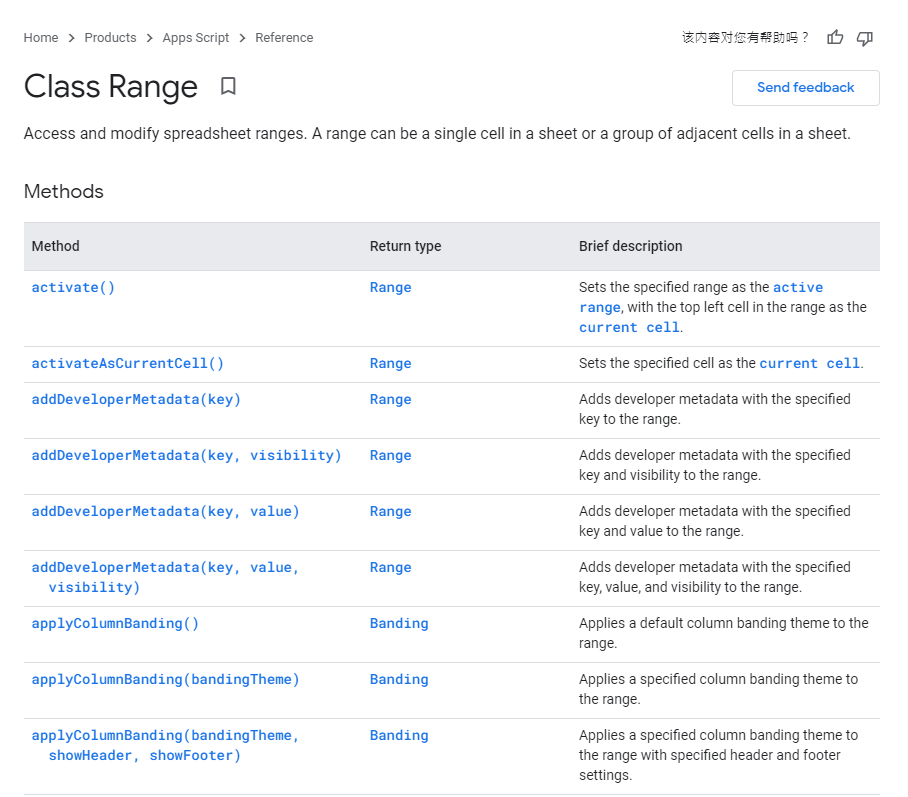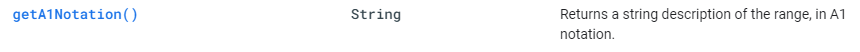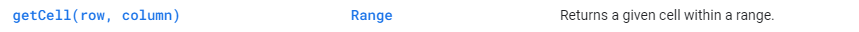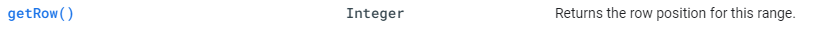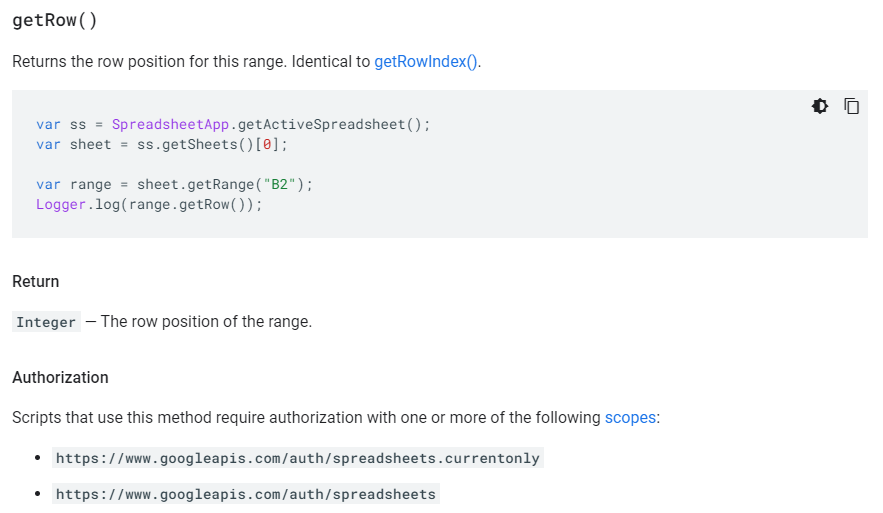``````function onEdit(e) {
console.log(`取得 \${e.range.getRow()} 行，該 A1 Notation 為 \${e.range.getA1Notation()}`);
}
``````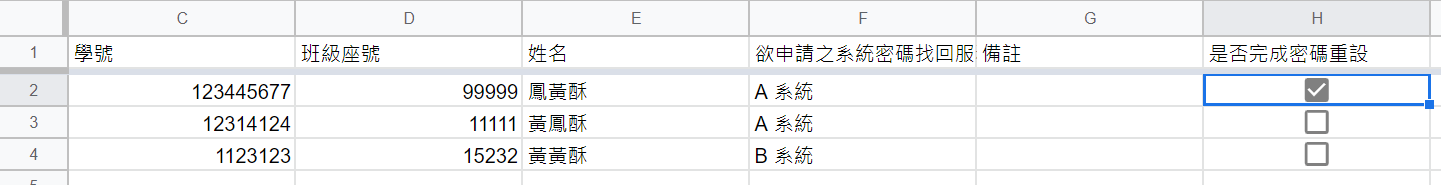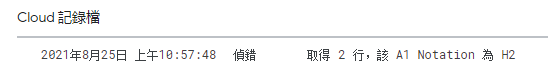Ok！這樣我們就能很簡單的取得需要的資訊，所以把程式完整一下：

``````function onEdit(e) {
const theRow = e.range.getRow();
const theData = {};
theData.email = e.range.getCell(theRow, 2);
theData.schoolId = e.range.getCell(theRow, 3);
theData.class = e.range.getCell(theRow, 4);
theData.name = e.range.getCell(theRow, 5);
console.log(theData);
}
``````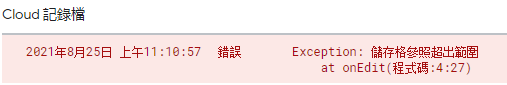``````function onEdit(e) {
const theSheet = e.range.getSheet();
const theRowIndex = e.range.getRow();
const theColIndex = e.range.getColumn();
const thisRange = theSheet.getRange(theRowIndex, 1, 1, theColIndex);
const theData = {}
theData.email = thisRange.getCell(1, 2);
theData.shoolId = thisRange.getCell(1,3);
theData.class = thisRange.getCell(1,4);
theData.name = thisRange.getCell(1,5);
console.log(theData);
}
``````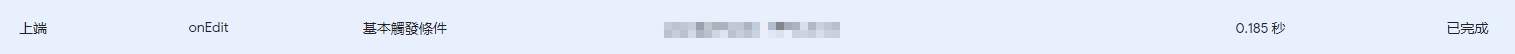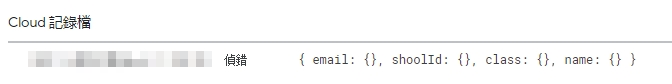……是怎樣，為什麼又沒有數值阿?‍♂️?‍♂️?‍♂️?‍♂️?‍♂️

``````function onEdit(e) {
const theSheet = e.range.getSheet();
const theRowIndex = e.range.getRow();
const theColIndex = e.range.getColumn();
const thisRange = theSheet.getRange(theRowIndex, 1, 1, theColIndex);
const theData = {}
theData.email = thisRange.getCell(1, 2).getValue();
theData.shoolId = thisRange.getCell(1,3).getValue();
theData.class = thisRange.getCell(1,4).getValue();
theData.name = thisRange.getCell(1,5).getValue();
console.log(theData);
}
``````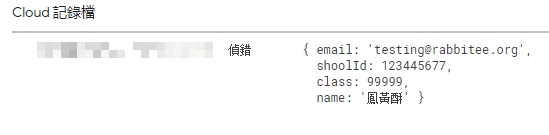### 關於兔兔們：``````學生：……

``````